# AP Calculus BC Review: Euler’s Method

What is Euler’s Method? In this post we review this technique for approximating solutions to certain kinds of differential equations and work out a few examples based on what you may see on the AP Calculus BC exam.

## Euler’s Method

Euler’s Method is a step-based method for approximating the solution to an initial value problem of the following type.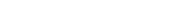The given time t0 is the initial time, and the corresponding y0 is the initial value.

First, you must choose a small step size h (which is almost always given in the problem statement on the AP exam).

Then the solution at later times t1, t2, t3, … can be found using the following algorithm.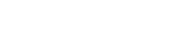### Why Does It Work?

It’s helpful to understand a little of the theory behind Euler’s Method. In fact, a good grasp of the theory helps you in other areas of calculus as well.

The key is that the y ‘ always measures the rate of change of the function y. So even if you don’t know what y is, if you can tell how fast it’s changing, then that info can help you to build up the values of y.

Now if y changes from y0 to y1 in the time interval from t0 to t1, then we can express this fact using the derivative: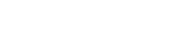Letting the difference in time be h = t1t0, then the formula becomes:The final piece of the puzzle is to use the given information that y ‘ = f(t, y).

That means that you can replace y ‘ by its current value as determined by the function f. If you’re only going from t0 to t1 (a single step), then you get: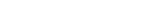On the other hand, why stop at just one step? Once you know the value of y1, then you can use that value in a similar way to find out y2. Then use y2 to find y3, etc.Using Euler’s Method is like detective work. One clue (y-value) leads you to the next.

### When Should I Use Euler’s Method?

It’s important to realize that this method does not give exact answers — just good estimates.

Unless the directions specify to use Euler’s Method, do not use it!

Instead, if you have to solve a differential equation or initial value problem, then try using methods like separation of variables or in the simplest cases, integration (for a refresher, take a look at: AP Calculus Exam Review: Integrals).

## Examples

Ok enough theory. Let’s get to the examples!

### Example 1 — Getting the Basics Down

Use Euler’s Method with h = 0.25 to estimate y(1) if y ‘ = 3y – 1 and y(0) = 1.

#### Solution

The first clue is in the given information. The equation y(0) = 1 means that the initial time is x0 = 0 and initial value is y0 = 1.

Note, here we are using x instead of t because sometimes the AP Calculus question will be in terms of x and y rather than t and y.

Because the step size is 0.25, we use xn = x0 + nh to get:Now remember, the derivative is given you you: y ‘ = 3y – 1. Use this to fill out the table.

nxnynyn'
0013(1) - 1 = 2
10.251 + 0.25(2) = 1.53(1.5) - 1 = 3.5
20.51.5 + 0.25(3.5) = 2.3753(2.375) - 1 = 6.125
30.752.375 + 0.25(6.125) = 3.9063(3.906) - 1 = 10.718
413.906 + 0.25(10.718) = 6.586

Finally, we see that our final answer is: y(1) = y4 = 6.586.

### Example 2 — Slightly More Challenging

Let y = f(t) be a function satisfying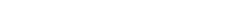. Use Euler’s Method with step size 0.5 to estimate the value of f(1).

#### Solution

Here, the step size is h = 0.5, so there will only be two new time values before landing on the goal time t = 1:

t1 = 0 + 1(0.5) = 0.5, and t2 = 0 + 2(0.5) = 1.

Also, the given derivative function looks messy. Just substitute y for f(t) to make it easier to work with.

So we can rephrase the question with y ‘ = t(2 – y). Notice this time the formula involves both variables, t and y in this case.

The initial value is y0 = 8.

ntnynyn'
0080(2 - 8) = 0
10.58 + 0.5(0) = 80.5(2 - 8) = -3
218 + 0.5(-3) = 6.5

### Example 3 — One More For the Road

Find y(2) by Euler’s Method with step size 0.2 if y(1) = 4 and y ‘ = y.Forgot your calculator? Maybe this abacus will help.

#### Solution

This time, with step size h = 0.2, there will be five new t-values to worry about.

ntnynyn'
0144
11.24 + 0.2(4) = 4.84.8
21.44.8 + 0.2(4.8) = 5.765.76
31.65.76 + 0.2(5.76) = 6.9126.912
41.86.912 + 0.2(6.912) = 8.2948.294
528.294 + 0.2(8.294) = 9.953

The preceding problem can be done by exact means as well. This will serve as a good verification that our method actually works.

If y ‘ = dy/dt = y, then by separation of variables, we have: (1/y) dy = dt.

Integrating both sides, we get: ln |y| = t + C.

Now because the initial value 4 is positive, we can safely ignore the absolute value bars in this problem. The use your algebra skills and the given initial values to solve for y.You can check on your own that plugging in t = 1 results in a value very close to 4. But what we’re really interested in is t = 2.

1.4715e2 = 10.873.

Even though our estimate of 9.953 seems off the mark compared to the actual result of 10.873, it’s actually not bad. In fact, better estimates could have been produced by decreasing the step size. At any rate, our estimate is in the same ballpark, which is all that can be hoped for when using Euler’s Method.

## Final Thoughts

While Euler’s Method may at first seem alien, it’s actually easy to use. You just have to plug the right numbers into a certain formula multiple times until you arrive at the desired result.

Conceptually, the method is not much different than tracing a path through a slope field. At each point, the direction of your path is determined by the direction of the arrows in the field just as the given function y ‘ = f(t, y) determines the values of y for later times t.Slope field for y ‘ = x + y

For a guide to understanding this and other topics on the AP Calculus BC test, start here: What to Expect on the AP Calculus BC Test.

#### Improve your SAT or ACT score, guaranteed. Start your 1 Week Free Trial of Magoosh SAT Prep or your 1 Week Free Trial of Magoosh ACT Prep today!## Author

•Shaun earned his Ph. D. in mathematics from The Ohio State University in 2008 (Go Bucks!!). He received his BA in Mathematics with a minor in computer science from Oberlin College in 2002. In addition, Shaun earned a B. Mus. from the Oberlin Conservatory in the same year, with a major in music composition. Shaun still loves music -- almost as much as math! -- and he (thinks he) can play piano, guitar, and bass. Shaun has taught and tutored students in mathematics for about a decade, and hopes his experience can help you to succeed!